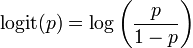## Thursday, January 13, 2011by Andrew Foland
Suppose that you are in possession of a nugget of knowledge that a team will win with probability p. The question, “What do you believe the probability of winning is?” is a very easy one to answer: p!
However, suppose you are in possession of two nuggets of knowledge. One nugget indicates that the probability of the team winning is p. The other nugget indicates, wholly independently, that the probability of the team winning is q. Now, if you are asked, “What do you believe the probability of this team winning is?”, the question is not as obvious to answer.

What is the best way to combine the information from p and q? (It is not, incidentally, to average the two!) Put another way, what should the formula f(p,q) be that creates the best estimate from the two?

Let’s first figure out some properties we think this formula should have:
1. If one of the pieces of information is “50/50”, then the estimate should simply be equal to the other piece of information. Knowing “50/50” on a binary win/loss outcome does not add information.
2. If q=1-p, then the probability should be 0.5 . Having q=1-p means that, for instance, one nugget says 25%, the other says 75%. In this case, the information should “cancel out” and leave you back with 50/50.
3. If either p or q is 100%, and the other does not equal 0%, the final probability should be 100%
4. If either p or q is 0%, and the other does not equal 100%, the final probability should be 0%
5. It shouldn’t matter if you learned p first or q first.
6. The final probability cannot exceed 1 or fall below 0.

It would make an interesting journal article or math graduate master’s thesis to determine the uniqueness of the functions which satisfy these conditions. (If you or your student ever do this, I want to be on the committee!) However, you can check for yourself that if you use the rule

logit(f)=logit(p)+logit(q)

then it will satisfy the above conditions. The logit function is the same function that Brian uses to generate the GWP; it is well described and defined here. In fact, consider p is the GWP of the one team, and q is (1-GWP) of the other team. Then our above considerations translate into
1. If either team has a GWP of 0.5, it is a generic team, and the other team’s GWP applies.
2. If both teams have equal GWP’s, each has an equal chance of winning
3. If one team’s GWP is 100%, it always wins games, regardless of the other team’s GWP, unless the other teams’ GWP is also 100%.
4. If one team’s GWP is 0%, it always wins games, regardless of the other team’s GWP, unless the other teams’ GWP is also 0%.
5. All GWP’s are created equal
6. You cannot have over 100% chance to win, or under 0% chance.

Note that while the logit formula can be used to combine two opposing GWP’s (by using 1-GWP of the opposition for q), it can also be used to combine two independent pieces of information about a single team’s probability to win.
I leave it as an exercise to the reader to demonstrate that the logit formula logit(f)=logit(p)+logit(q) given above algebraically leads to the following probability formula in terms of p and q:

f=pq/[pq+(1-p)(1-q)]

Some of you may recognize this as a version of Bill James’s “Log5 Rule” for combining team winning percentages. There is also an intuitive (if slightly handwavey) way to describe this last formula. Either you win or you don’t. Winning means that you hit both p and q; the probability of that is found by multiplying pq. Losing means you hit neither of p nor q; the probability is again the product, (1-p)(1-q). The total probability is [pq+(1-p)(1-q)], and the fraction of that due to winning is pq, so the total win fraction is pq/[pq+(1-p)(1-q)].

(Even if you don’t entirely buy this line of argument, it is nonetheless the case that this formula satisfies the conditions we want it to, and is therefore the formula we are looking for.)